Clutch Prep is now a part of Pearson
Ch.11 - EDTA TitrationsWorksheetSee all chapters

# EDTA

See all sections
Sections
Metal Chelate Complexes
EDTA
EDTA Titration Curves
###### EDTA

Concept #1: EDTA represents the most versatile polydentate compound that can be used in numerous titrations to determine unknown concentrations for reacting metal ions.

EDTA represents a hexaprotic compound that possesses 7 different forms based on the pH of the solution.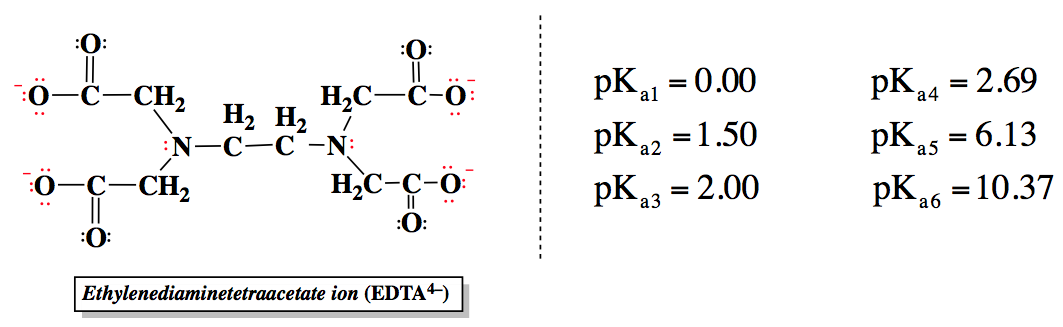The basic form of EDTA, Y4-, is the predominate form at higher pH values, while the acidic form, H6Y2+, predominates at lower pH values.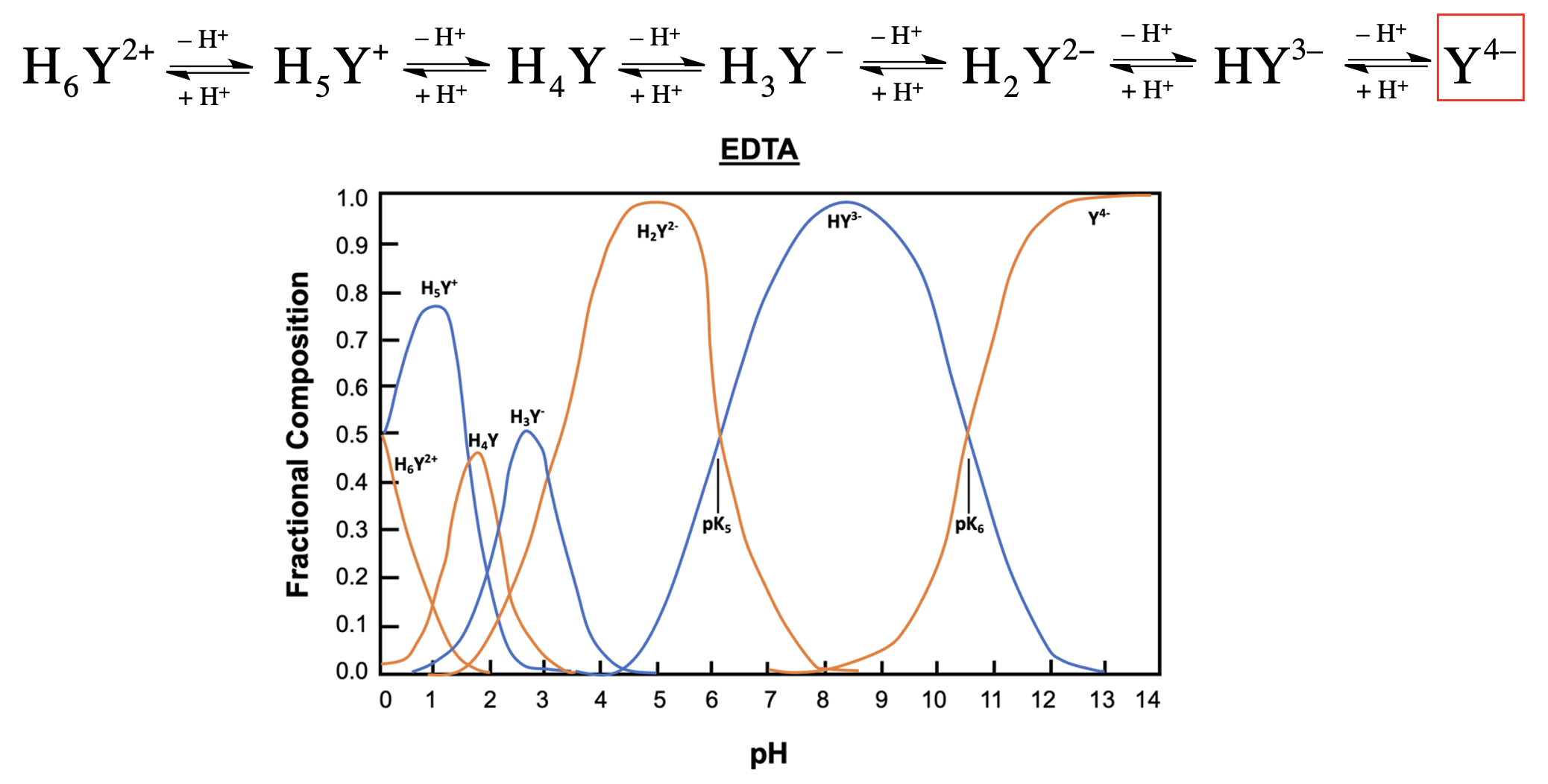The fraction of basic form present in solution can be determined by either given concentrations or by the pH of the solution.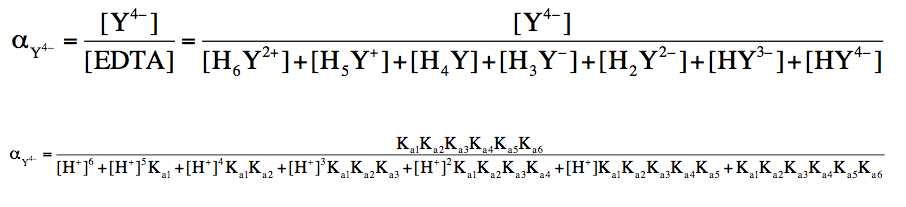###### EDTA Calculations

Example #1: The formal concentration of EDTA is 1.50 mM. What is the concentration of the Y4 – form at a pH of 5.0?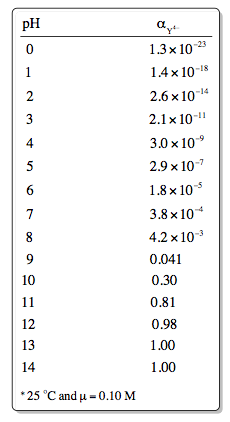Example #2: Determine the αY4- for EDTA when the pH = 8.50.

Practice: Determine the pH where αY4– equals 0.20.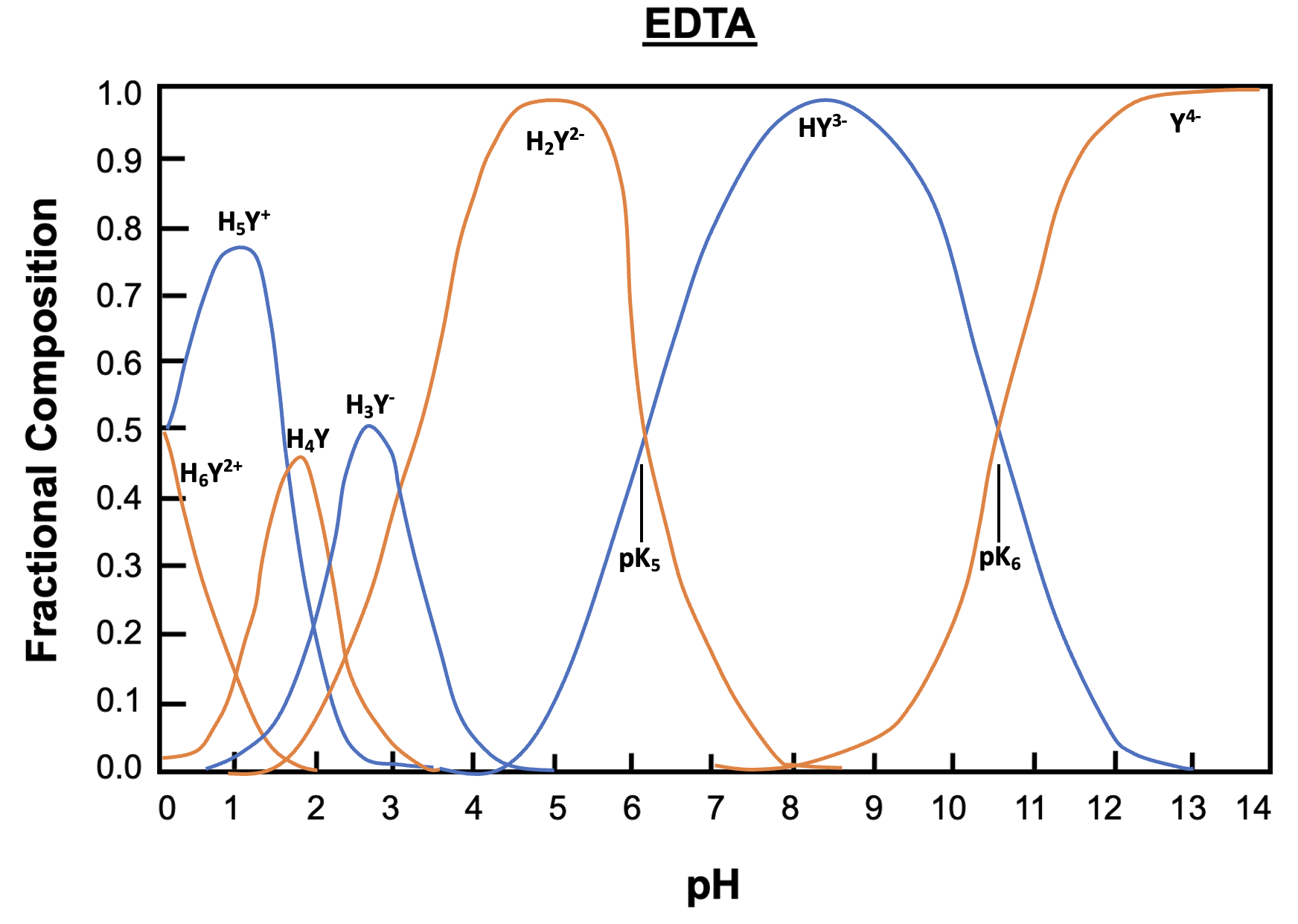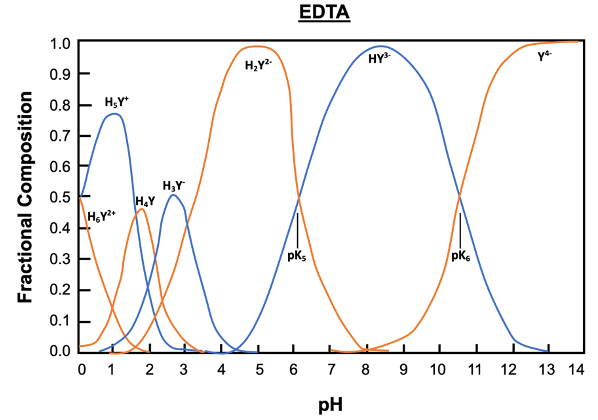###### EDTA Complexes

Concept #2: The stability or formation constant is used when an equilibrium is established between a ligand and a metal, while the conditional formation constant is used when our solution is buffered to a specific pH.

Each metal ion has its own unique formation constant.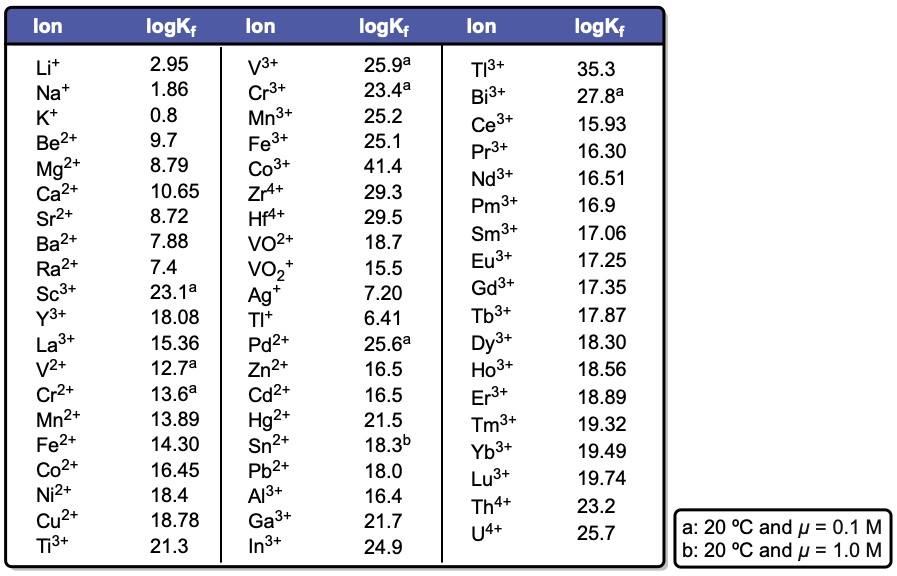The conditional formation constant can be calculated by the determining the fraction of the basic form and the formation constant of the metal ion.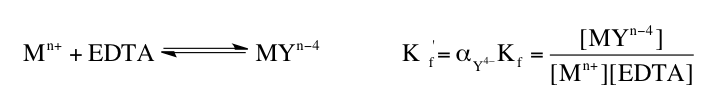Example #3: Find [Ba2+] in 0.10 M BaY2- at pH = 10.00.

###### EDTA Complexes Calculations

Example #4: Determine the conditional formation constant for Co(EDTA) at pH = 8.00.

Example #5: Find the concentration of free Sn2+ in 0.20 M K2[Sn(EDTA)] at pH = 9.00.

Practice: Find the concentration of free Na + in 0.15 M Li 3[Na(EDTA)] at pH = 10.00.